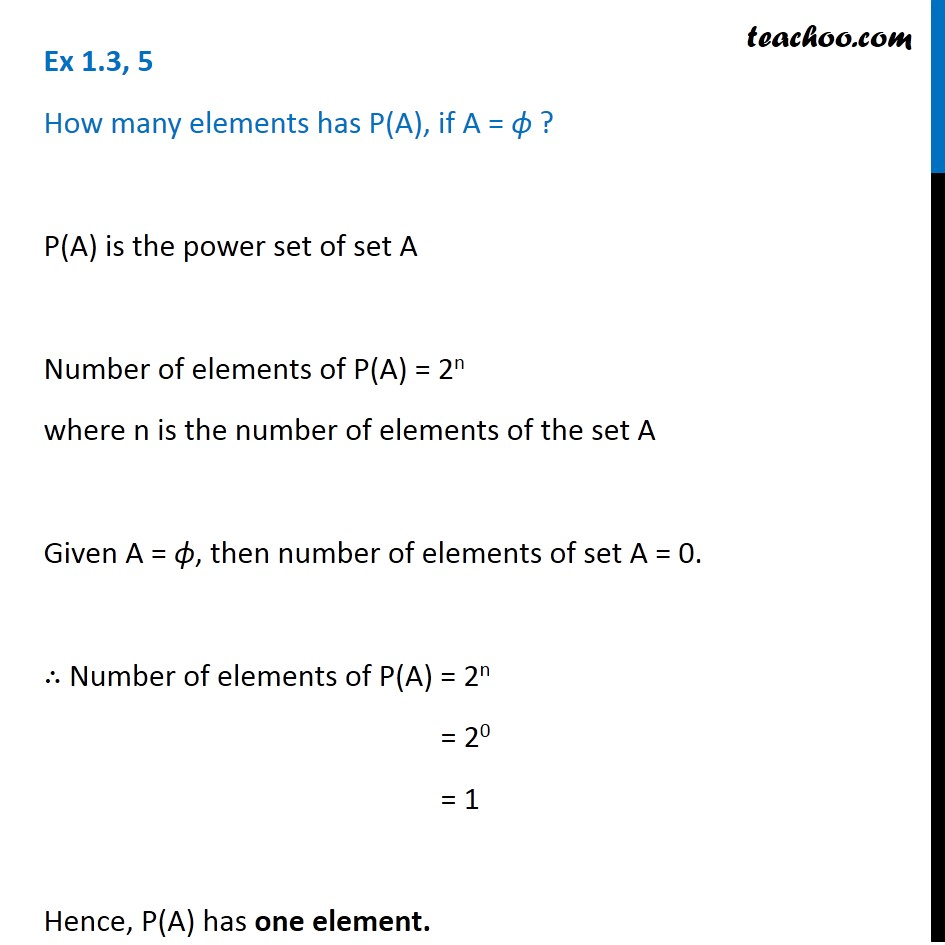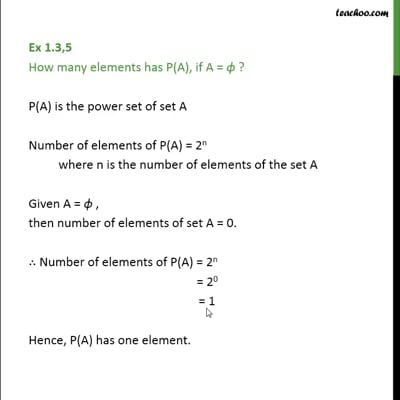Ex 1.3

Chapter 1 Class 11 Sets
Serial order wiseThis video is only available for Teachoo black users

Learn in your speed, with individual attention - Teachoo Maths 1-on-1 Class

### Transcript

Question 1 How many elements has P(A), if A = ϕ ? P(A) is the power set of set A Number of elements of P(A) = 2n where n is the number of elements of the set A Given A = ϕ, then number of elements of set A = 0. ∴ Number of elements of P(A) = 2n = 20 = 1 Hence, P(A) has one element.